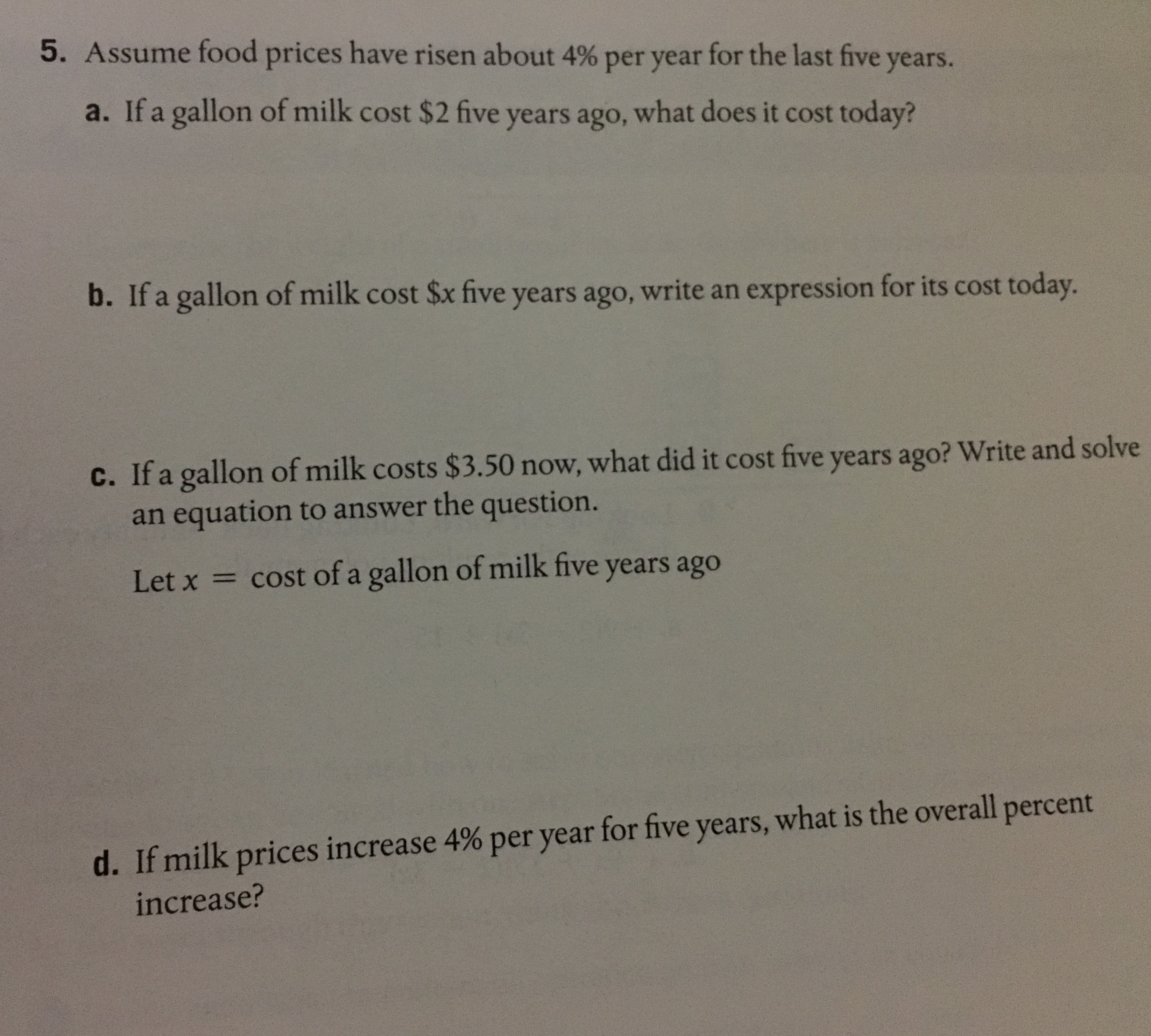# 5. Assume food prices have risen about 4% per year for the last five years.a. If a gallon of milk cost \$2 five years ago, what does it cost today?b. If a gallon of milk cost Sx five years ago, write an expression for its cost today.c. If a gallon of milk costs \$3.50 now, what did it cost five years ago? Write and solvean equation to answer the question.Let xcost of a gallon of milk five years agod. Ifmilk prices increase 4% per year for five years, what is the overall percentncrease

Questionhelp_outlineImage Transcriptionclose5. Assume food prices have risen about 4% per year for the last five years. a. If a gallon of milk cost \$2 five years ago, what does it cost today? b. If a gallon of milk cost Sx five years ago, write an expression for its cost today. c. If a gallon of milk costs \$3.50 now, what did it cost five years ago? Write and solve an equation to answer the question. Let x cost of a gallon of milk five years ago d. Ifmilk prices increase 4% per year for five years, what is the overall percent ncrease fullscreen
check_circle

Step 1

Thank you for the question. Here there are 4 subparts in the question. According to our policies cannot answer more than 3 questions per session. So I am answering first three questions. You can use these solutions as reference and solve last part or you can request for help through new request.

Step 2

Given that food prices risen about 4% per year for the last five years.

Given that gallon of milk cost \$2 five years ago, we need to find its cost today.

If a quantity a0 is increased by i% every year and then after n years it is accumulated to an. then an is given by the below mentioned formula and we know here given that every year 4% increase in price of milk per gallon, so i = 4% and five years ago price is \$2. So a0=2. Here we need to find the price after 5 years so n = 5. By substituting this values we can calcualte the price of milk after 5 years.

We get price of gallon of milk after 5 years if the price is raising from 2\$ at 4% per year is \$2.43 (after rounding upto two decimals).

Step 3

Given a gallon of milk cost x\$ five years ago and it increases at rate 4% per year for the last five years.

If a quantity a0 is increased by i% every year and then after n years it is accumulated to an. then an is given by the below mentioned formula and here given that every year 4% increase in price of milk per gallon, so i = 4% and five years ago price is \$x. So a0...

### Want to see the full answer?

See Solution

#### Want to see this answer and more?

Solutions are written by subject experts who are available 24/7. Questions are typically answered within 1 hour.*

See Solution
*Response times may vary by subject and question.
Tagged in

### Algebra